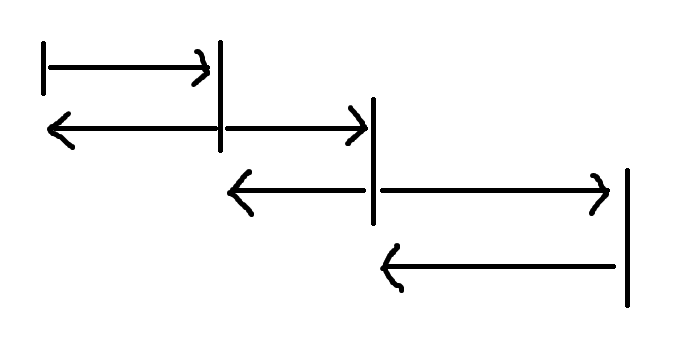# 如果我不知道把一些东西放在哪里，那就放在这里吧

## 实际上是一些trick

Posted by ShanLunjiaJian's Blog on November 16, 2021

(gf)不知道干什么的时候你就求个导。

(任何情况下)不知道干什么的时候你就打个表。

(线性规划)不知道干什么的时候你就对偶一下。

(数数)不知道干什么的时候你就容斥一下。

(数数)不知道干什么的时候你就看看是不是独立的。

(任何情况下)不知道干什么的时候你就枚举一个方向。

(主要是ds)不知道干什么的时候你就把时间维画出来。

(博弈论)不知道干什么的时候你就考虑一个胜负确定的无穷集合。

make hld great again!

• 第一遍从上到下分配重链。

• 第二遍从上到下分配重链上每个点的所有轻儿子。这导致除了以$1$为根的链，别的链在第一步的时候，链头是已经分配完了的，它和重链剩下的部分不连续，需要特殊处理。

• 第三遍从上到下递归重链上每个点的所有轻儿子的重链。

how it works?

• 对于链，显然。

• 对于毛毛虫，每次往上跳需要考虑一条重链的一个区间的所有点和它们的所有轻儿子，这是两个标号区间。注意如果信息不幂等，需要换一种对链头的特殊处理(实际上就是不把它算到这条重链里，除了在lca处特判)，因为它会作为它父亲的一个轻儿子。

• 对于子树，考虑这个点所在的重链从它开始往下的部分。它的子树分为这半条重链，这半条重链上每个点的轻儿子，这半条重链上每个点的轻子树，而三部分分别是一个标号区间。

Prufer序列

$n$个点的有标号无根树和长$n-2$值域在$1,…,n$的序列形成双射。每个点在序列中的出现次数是它的度数$-1$。

Pick定理

lxl口中的经典题

diamond计数

diamond指的是一个四元环加上一条弦。Stern-Brocot Tree

s-b树的发现本来就是用来二分有理数的，它的独立发现者之一Brocot就是一名钟表匠。比如如果你有两个齿轮在一根轴上，一个接在电机上而另一个接在指针，你希望这两个齿轮可以把电机的转速变成秒针的转速。齿数显然都必须是整数，于是我们需要用有理数来拟合一个实数。并且这个齿数不能过大，也就是说拟合的时候分子分母都不能过大，于是s-b树这种二分有理数的工具就诞生了。在Pell方程普及前，s-b树仅有的板子题就是divcnt1，它是一个$\tilde{O}(\sqrt{n})$求$d$或者说$\sigma_0$的前缀和的算法的一部分。

s-b树只包括正有理数，如果加入负的，我们得到s-b环(stern-brocot wreath)(从$\frac{0}{1},\frac{1}{0},\frac{0}{-1},\frac{-1}{0},\frac{0}{1}$开始)，它表示平面上所有有理方向。

s-b树上两个点lca的意义是，它俩之间分母最小的数。

s-b树还有奇怪的几何意义，可以搜索 Ford Circle。

loj3401

ioi2018 排座位

$v-e=1$的技巧大家都知道!

Konig定理

Hall定理

• 一条边$u\rightarrow v$可行当且仅当这条边满流，并且残量网络上不存在$u\rightsquigarrow v$的路径。如果存在$u\rightsquigarrow v$的路径，那么割掉它之后这条路径就是增广路了，于是这条路径应该也满流，但是它没满流。

• 一条边$u\rightarrow v$必经当且仅当这条边满流，并且残量网络上存在$s\rightsquigarrow u$的路径和$v\rightsquigarrow t$的路径。容易感觉到此时这条边不得不割，并且如果不存在这样的路径的话，这条边就可以不割了，因为说明前面或者后面有一些充满了的边，可以割那些边来代替这一条。

quick hull，以及某种奇怪的lambda技巧

quick hull的经典用法是最小乘积生成树。最小乘积生成树说的是每个点有两个权值$a,b$，求$\left\sum a\right\left\sum b\right$最小的生成树。

$$\mathcal{A}=\mathrm{SEQ}(\mathcal{B})\Rightarrow A(z)=\displaystyle\frac{1}{1-B(z)}$$ 请注意你可以，或者说应当把$\displaystyle\frac{1}{1 − B(z)}$看成$1+B(z)+B^2(z)+B^3(z)+…$的简写。

——x义x《组合对象符号化·构造组合类·Sequence构造》

$d(n)$有多大?

$O(2^{\frac{(1+o(1))\ln n}{\ln\ln n}})$。离谱。

usaco21Feb Ag Just Green Enough

trie上的子树

tutte矩阵

kru

kru的作用是，根据生成森林构成拟阵的性质，把最小生成森林转化成 加入一条边之后是不是还是生成森林。做pjudge R1 B(2021-2022 ICPC North America Championships. Problem I)的时候见识到了。

$\frac{1}{2^nn!}\sum_{p\in S_{2n}}\mathrm{sgn}(p)\prod_{i=1}^na_{p_{2i-1},p_{2i}}$

$\varphi(ij)=\frac{\varphi(i)\varphi(j)\gcd(i,j)}{\varphi(\gcd(i,j))}$ $\mu^2(n)=\sum_{d^2\mid n}\mu(d)$

bzoj3774

2018-2019 ACM-ICPC, Asia East Continent Finals B. Mysterious … Host

sdsc2020(sdptt) D4 C. geometry

Ore定理

Posa定理

Bondy-Chvatal定理

\begin{aligned} x\operatorname{or}(y\operatorname{and}z)&=(x\operatorname{or}y)\operatorname{and}(x\operatorname{or}z)\\ x\operatorname{and}(y\operatorname{or}z)&=(x\operatorname{and}y)\operatorname{or}(x\operatorname{and}z)\\ x\operatorname{and}(y\operatorname{xor}z)&=(x\operatorname{xor}y)\operatorname{and}(x\operatorname{xor}z) \end{aligned}

fuck myself

• 最小(权)覆盖 : 用最少(权值和最小)的线段覆盖某个给定区间

• 最大(权)独立集 : 选出最多(权值和最大)的不交线段

。这两个是经典的最短路建图。

dp，设$dp(i)$表示最右边的线段是$i$的答案，那么右端点在这条线段的范围内，而前面的部分需要和前面的状态接起来，用线段树维护区间max来转移。

dp，设$dp(i)$表示最右边的线段是$i$的答案，那么右端点在这条线段的右边，而前面的部分需要从前面的状态转移过来，可以直接维护前缀max。

1
2
3
4
5
6
7
8
9
10
inline int find(int m)
{
int now=0,c=0;
for(int i=1;i<=n;i++)
if(a[i]==now) c++;
else
if(c==0) c=1,now=a[i];
else c--;
return c>0?now:-1;
}


1
2
3
4
5
6
7
8
9
10
11
12
13
14
15
16
17
18
19
inline int find(int m)
{
int now=0,c=0,c1=0,ct=0,t=-1;
for(int i=1;i<=n;i++)
{
if(a[i]==now) c++;
else
if(c==0) c=1,now=a[i];
else
{
c--;
if(t==-1) t=a[i];
}
c1+=(a[i]==b),ct+=(a[i]==t);
}
if(c>0) return now;
if(c1*2>=n) return a;
if(ct*2>=n) return t;
}


hash

sd省选，爆力打满就进了。A和B差不止一题，但是进和不进只差爆力。

laofu 模拟费用流 的 Problem 2

• 如果遇到了一个老鼠，两边分别全局加

• 如果遇到了一个洞，决策选不选

• 如果之前剩下了老鼠，那么必然选它，这是显然的

• 如果之前啥也没有，需要决策一下

• 如果之前剩下了洞，那么必然选它。考虑对于任何一个策略，$\vert j\vert$看起来是若干段斜率为$1,-1,0$之一的折线。我们以这条折线中在$x$轴上的点作为分隔，把折线分成若干段，那么这个决策方法说的是，我们所做的就是决策每个以洞开始的段何时开始，然后这一段就一直选下去直到回到$x$轴上。为了证明正确性，我们只需要说明每一个以洞开始的段，一旦开始，则在它结束之前，一直选下去是最优的。考虑如果一段之中，我们中间某个洞没有选，那么把选第一个洞换成选中间这个洞必然更优。因为这是同一段，中间不会触碰到$x$轴，这个交换所做的就是把两个洞之间这一段下移了一个单位长度，所以它仍然合法。同时在由洞开始的一段中，洞的贡献是负的坐标，所以把前面换成后面答案会更小。

$\vartheta^n=\sum_{k=0}^n{n\brace k}z^k\mathrm D^k$

，当用它搞$n^k$时，对应于把$n^k$展开成下降幂，这可以在下面这个题中体现。

$\sum_{k=0}^n\binom{n}{k}x^kf(k)$

$\sum_{k=0}^n\binom{n}{k}k^tx^k$

\begin{aligned} &\sum_{k=0}^n\binom{n}{k}k^tx^k\\ =&\sum_{k=0}^n\binom{n}{k}\vartheta^t x^k\\ =&\vartheta^t\sum_{k=0}^n\binom{n}{k}x^k\\ =&\sum_{i=0}^t{t\brace i}\sum_{k=0}^n\binom{n}{k}k^{\underline{t}}x^k\\ =&\sum_{i=0}^t{t\brace i}\sum_{k=0}^n\frac{n^{\underline{k}}}{k!}k^{\underline{t}}x^k\\ =&\sum_{i=0}^t{t\brace i}\sum_{k=0}^nn^{\underline{t}}\frac{(n-t)^{\underline{k-t}}}{(k-t)!}x^k\\ =&n^{\underline{t}}\sum_{i=0}^t{t\brace i}\sum_{k=0}^n\binom{n-t}{k-t}x^k\\ =&n^{\underline{t}}x^t\sum_{i=0}^t{t\brace i}\sum_{k=0}^{n-t}\binom{n-t}{k}x^k\\ =&n^{\underline{t}}x^t\sum_{i=0}^t{t\brace i}(1+x)^{n-t}\\ \end{aligned}

• $4K_1$ : 容斥系数是$1$。

• $2K_1+K_2$ : 考虑它里面有一个$4K_1$，所以它的容斥系数应该是$-1$。

• $K_1+P_3$ : 考虑它里面有一个$4K_1$，两个$2K_1+K_2$，所以它的容斥系数应该是$1$。

• $K_1+K_3$ : 考虑它里面有一个$4K_1$，三个$2K_1+K_2$，三个$K_1+P_3$，所以它的容斥系数应该是$-1$。

• ……

$\sum_u\sum_{v\in\mathrm{ch}(u)}\min(\mathrm{size}(u)-\mathrm{size}(v),k)\min(\mathrm{size}(v),k)=O(nk)$

，比如花老师在vuq提到qoj4815 ptzsc22 D3 F. Flower’s Land。

Induction puzzle, Muddy children puzzle

1
2
3
4
5
6
7
8
9
M _calc(long long p,long long q,long long r,long long n,M U,M R)
{
if(!l) return M();
if(p>=q) return _calc(p%q,q,r,n,U,pow(U,p/q)*R);
long long m=((__int128)p*n+r)/q-1;
if(m==-1) return pow(R,n);
return pow(R,(q-r-1)/p)*U*_calc(q,p,(q-r-1)%p,m,R,U)*pow(R,n-((__int128)q*m+r)/p);
}
M calc(long long p,long long q,long long r,long long n,M U,M R){ return pow(U,r/q)*_calc(p,q,r%q,n,U,R); }


• 如果$j$更小，我们知道$1,…,i-1$中$j$是最小的，而$j,j+1,…j+k$分别比$i,i+1,…,i+k$小，所以可以直接$i:=i+k+1$。

• 如果$i$更小，我们知道$i,i+1,…i+k$分别比$j,j+1,…,j+k$小，所以可以直接$i:=j+k+1$。

dfa minimization

lovasz local lemma

$\mathrm{Pr}(A)\leq x_A\prod_{B\in\Gamma(A)}(1-x_B)$

$\mathrm{Pr}(A)\leq (1-\epsilon)x_A\prod_{B\in\Gamma(A)}(1-x_B)$

entropy compress

block design，有限射影平面

• 两个点确定一条线。

• 两条线有恰好一个交点。

• 存在四个点，使得没有一条线经过其中至少三个。这个条件用来排除一些平凡(但是也许离奇)的情况，比如所有点都在一条线上。

Mertens定理

Mertens定理说的是$\prod\limits_{p\leq n}\left(1-\frac{1}{p}\right)=\frac{e^{-\gamma}}{\ln n}\left(1+O(\frac{1}{\log n})\right)=\Theta(\frac{1}{\log n})$，其中$e^{-\gamma}\approx 0.56$。

http://sweet.ua.pt/tos/gaps.html

Mertens猜想

$\mu$的前缀和很小。如果黎曼猜想成立，它将是$O(n^{\frac{1}{2}+\epsilon})$的，并且已经验证它在你会用到的范围内不会超过$\sqrt{n}$。有人猜测它是$O(\sqrt{n})$，有人认为不尽如此，你怎么看?

Pollock四面体数猜想指出，任意自然数可以表示成不超过五个四面体数$\binom{n+2}{3}$的和。只用四个，猜想只有有限个反例，它的反例在 http://oeis.org/A000797 ，已知最大的在3e5级别。

Pollock八面体数猜想指出，任意自然数可以表示成不超过七个八面体数$\frac{n(2n^2+1)}{3}$的和。这个好像就被研究的少一点。

Foata变换

fkt

$h_i=\sum_{2j\leq i}f_jg_{i-j}$

$\log/\log\log$叉分治，注意到每一层我们所做的dft总长是$n$。

$F^R=z^nF(\frac{1}{z})$，一般$n=\deg F$。它的经典作用是，考虑$F$的所有根，设为$a_1,…,a_n$，那么$F=\prod\limits_i(z-a_i)$，而$F^R=\prod\limits_i(1-a_iz)$，这个形式在部分分式的时候好像有其作用。

lucas要求$\binom{n}{k}\bmod{p}$，也就是$[z^k] (1+z)^n\bmod{p}$。

loj6142 加强版

ei指出的问题

xvii poi出现了把数分进两个栈的情况，此时变成，如果存在三个位置$i<j<k$，$a_k<a_i<a_j$，那么$i,j$不能在同一个栈中。